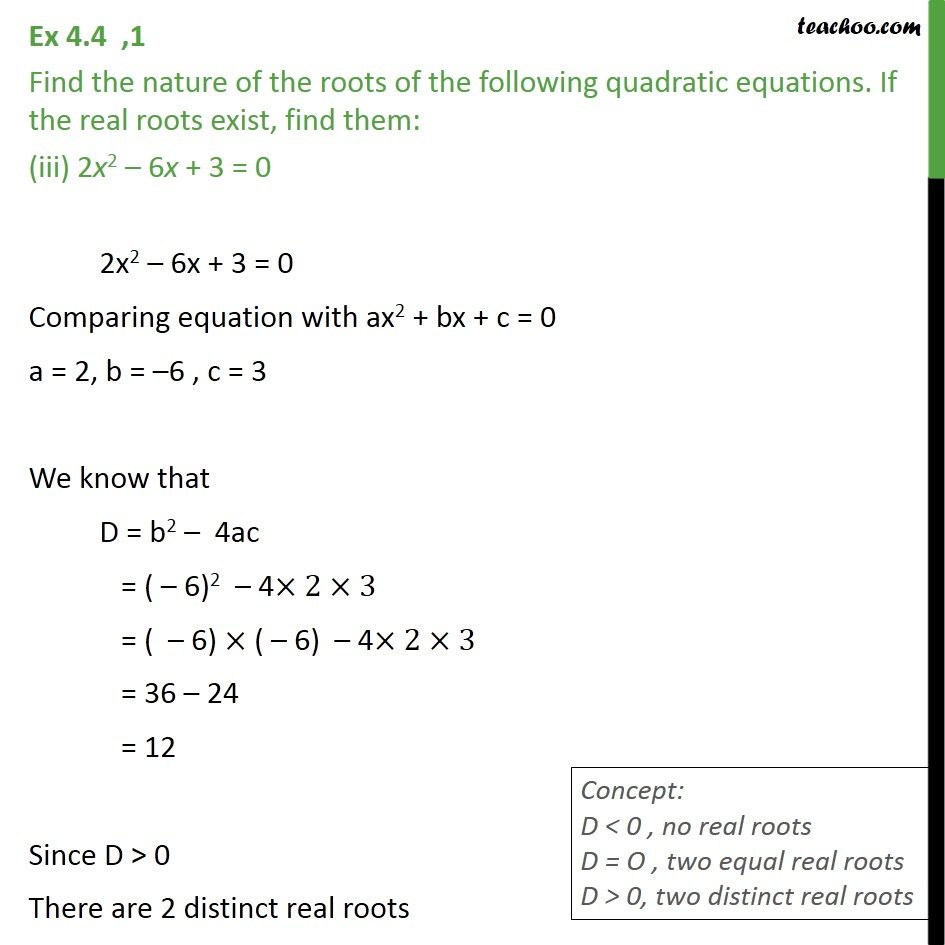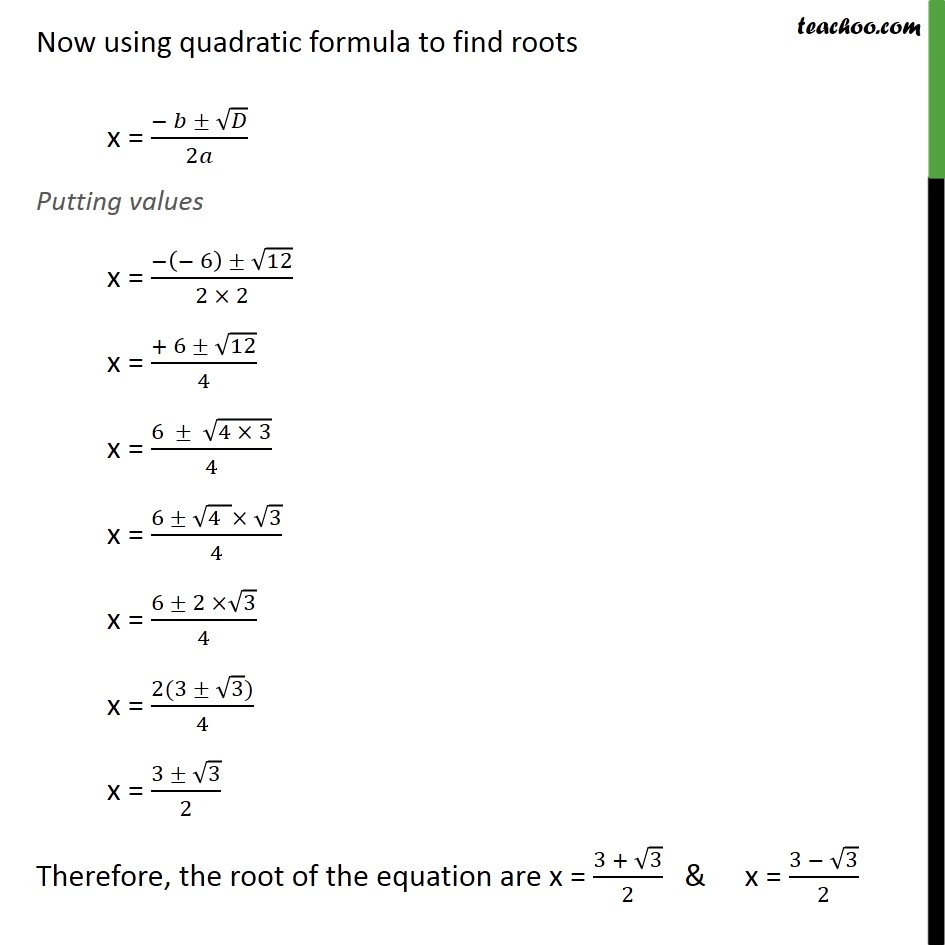Completing the square and Word Problems

Chapter 4 Class 10 Quadratic Equations
Serial order wiseLearn in your speed, with individual attention - Teachoo Maths 1-on-1 Class

### Transcript

Ex 4.3 ,1 Find the nature of the roots of the following quadratic equations. If the real roots exist, find them: (iii) 2x2 – 6x + 3 = 0 2x2 – 6x + 3 = 0 Comparing equation with ax2 + bx + c = 0 a = 2, b = –6 , c = 3 We know that D = b2 – 4ac = ( – 6)2 – 4×2×3 = ( – 6) × ( – 6) – 4×2×3 = 36 – 24 = 12 Since D > 0 There are 2 distinct real roots Now using quadratic formula to find roots x = (− 𝑏 ± √𝐷)/2𝑎 Putting values x = (−(− 6) ± √12)/(2 × 2) x = (+ 6 ± √12)/4 x = (6 ± √(4 × 3))/4 x = (6 ± √(4 )× √3)/4 x = (6 ± 2 ×√3)/4 x = (2(3 ± √3))/4 x = (3 ± √3)/2 Therefore, the root of the equation are x = (3 + √3)/2 & x = (3 − √3)/2